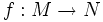# Sard's theorem

Suppose$M$ and$N$ are differential manifolds and$f:M \to N$ is a smooth map between them. Then, the set of regular values of$f$ is a subset of measure zero in$N$.
Suppose$M$ and$N$ are differential manifolds, and the dimension of$M$ is strictly less than the dimension of$N$. Then, if$f:M \to N$ is a smooth map, the image$f(M)$ has measure zero as a subset of$N$. In particular,$f$ cannot be surjective.